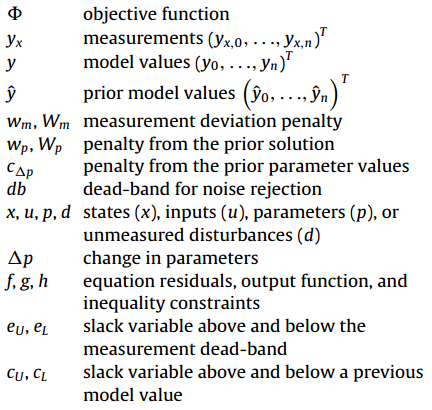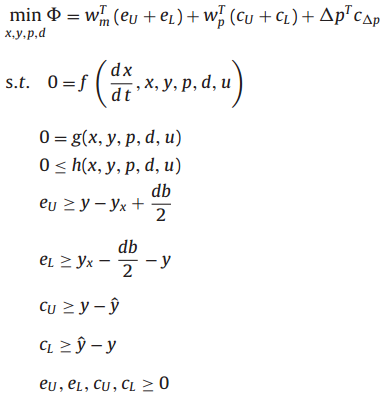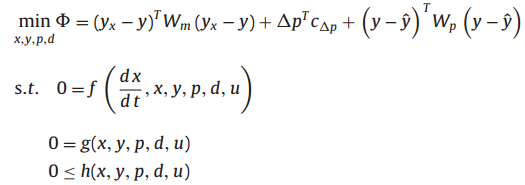## Main.EstimatorObjective History

March 19, 2019, at 02:56 PM by 10.35.117.63 -
Changed line 91 from:

The spread of HIV in a patient is approximated with balance equations on (H)ealthy, (I)nfected, and (V)irus population counts2.

to:

The spread of HIV in a patient is approximated with balance equations on (H)ealthy, (I)nfected, and (V)irus population counts2. Additional information on the HIV model is at the Process Dynamic and Control Course.

Changed line 67 from:

$$x,u,p=\mathrm{states,\,inputs,\,parameters}$$

to:

$$x,y,p=\mathrm{states,\,outputs,\,parameters}$$

Changed lines 39-41 from:

$$\Delta p_U \ge p - \hat p$$

$$\Delta p_L \ge \hat p - p$$

to:

$$\Delta p_U \ge p_i - p_{i-1}$$

$$\Delta p_L \ge p_{i-1} - p_i$$

Changed lines 11-12 from:

$$\min_{x,y,p} \Phi = \left(y_x-y\right)^T W_x \left(y_x-y\right) + \left(y-\hat y\right)^T W_m \left(y-\hat y\right) + \Delta p^T c_{\Delta p} \Delta p$$

to:

$$\min_{x,y,p} \Phi = \left(y_x-y\right)^T W_x \left(y_x-y\right) + \left(y-\hat y\right)^T W_m \left(y-\hat y\right) + \Delta p^T W_{\Delta p} \Delta p$$

Changed line 63 from:

$$c_{\Delta p}=\mathrm{parameter\,movement\,penalty\,(DCOST)}$$

to:

$$w_{\Delta p},W_{\Delta p}=\mathrm{parameter\,movement\,penalty\,(DCOST)}$$

Changed line 55 from:

$$y=\mathrm{\mathrm{model\,predictions}$$

to:

$$y=\mathrm{model\,predictions}$$

Changed lines 51-77 from:

$$\Phi = \mathrm{Objective\,Function}$$

$$y_x$$ = measurements

$$y$$ = model predictions

$$\hat y$$ = prior model values

$$w_x, W_x$$ = measurement deviation penalty (WMEAS)

$$w_m, W_m$$ = prior prediction deviation penalty (WMODEL)

$$c_{\Delta p}$$ = parameter movement penalty (DCOST)

$$db$$ = dead-band for noise rejection

$$x,u,p$$ = states, inputs, parameters

$$\Delta p$$ = parameter change

$$f,g$$ = equality and inequality constraints

$$e_U,e_L$$ = upper and lower error outside dead-band

$$c_U,c_L$$ = upper and lower deviation from prior model prediction

$$\Delta p_U,\Delta p_L$$ = upper and lower parameter change

to:

$$\Phi=\mathrm{Objective\,Function}$$

$$y_x=\mathrm{measurements}$$

$$y=\mathrm{\mathrm{model\,predictions}$$

$$\hat y=\mathrm{prior\,model\,values}$$

$$w_x, W_x=\mathrm{measurement\,deviation\,penalty\,(WMEAS)}$$

$$w_m, W_m=\mathrm{prior\,prediction\,deviation\,penalty\,(WMODEL)}$$

$$c_{\Delta p}=\mathrm{parameter\,movement\,penalty\,(DCOST)}$$

$$db=\mathrm{dead-band\,for\,noise\,rejection}$$

$$x,u,p=\mathrm{states,\,inputs,\,parameters}$$

$$\Delta p=\mathrm{parameter\,change}$$

$$f,g=\mathrm{equality\,and\,inequality\,constraints}$$

$$e_U,e_L=\mathrm{upper\,and\,lower\,error\,outside\,dead-band}$$

$$c_U,c_L=\mathrm{upper\,and\,lower\,deviation\,from\,prior\,model\,prediction}$$

$$\Delta p_U,\Delta p_L=\mathrm{upper\,and\,lower\,parameter\,change}$$

Changed line 51 from:

$$\Phi = \mathrm{Objective Function}$$

to:

$$\Phi = \mathrm{Objective\,Function}$$

Changed line 51 from:

$$\Phi$$ = Objective Function

to:

$$\Phi = \mathrm{Objective Function}$$

Changed lines 51-77 from:to:

$$\Phi$$ = Objective Function

$$y_x$$ = measurements

$$y$$ = model predictions

$$\hat y$$ = prior model values

$$w_x, W_x$$ = measurement deviation penalty (WMEAS)

$$w_m, W_m$$ = prior prediction deviation penalty (WMODEL)

$$c_{\Delta p}$$ = parameter movement penalty (DCOST)

$$db$$ = dead-band for noise rejection

$$x,u,p$$ = states, inputs, parameters

$$\Delta p$$ = parameter change

$$f,g$$ = equality and inequality constraints

$$e_U,e_L$$ = upper and lower error outside dead-band

$$c_U,c_L$$ = upper and lower deviation from prior model prediction

$$\Delta p_U,\Delta p_L$$ = upper and lower parameter change

Changed line 11 from:

$$\min_{x,y,p} \Phi = \left(y_x-y\right)^T W_m \left(y_x-y\right) + \Delta p^T c_{\Delta p} \Delta p + \left(y-\hat y\right)^T W_p \left(y-\hat y\right)$$

to:

$$\min_{x,y,p} \Phi = \left(y_x-y\right)^T W_x \left(y_x-y\right) + \left(y-\hat y\right)^T W_m \left(y-\hat y\right) + \Delta p^T c_{\Delta p} \Delta p$$

Changed lines 23-24 from:

$$\min_{x,y,p} \Phi = w_m^T \left(e_U+e_L\right) + w_p^T \left(c_U+c_L\right) + w_{\Delta p}^T \left(\Delta p_U+\Delta p_L\right)$$

to:

$$\min_{x,y,p} \Phi = w_x^T \left(e_U+e_L\right) + w_m^T \left(c_U+c_L\right) + w_{\Delta p}^T \left(\Delta p_U+\Delta p_L\right)$$

Changed lines 39-43 from:

$$e_U, e_L, c_U, c_L \ge 0$$

to:

$$\Delta p_U \ge p - \hat p$$

$$\Delta p_L \ge \hat p - p$$

$$e_U, e_L, c_U, c_L, \Delta p_U, \Delta p_L \ge 0$$

Changed line 23 from:

$$\min_{x,y,p} \Phi = w_m^T \left(e_U+e_L\right) + w_p^T \left(c_U+c_L\right) + \left| \Delta p \right|^T c_{\Delta p}$$

to:

$$\min_{x,y,p} \Phi = w_m^T \left(e_U+e_L\right) + w_p^T \left(c_U+c_L\right) + w_{\Delta p}^T \left(\Delta p_U+\Delta p_L\right)$$

Changed line 23 from:

$$\min_{x,y,p} \Phi = w_m^T \left(e_U+e_L\right) + w_p^T \left(c_U+c_L\right) + \Delta p^T c_{\Delta p}$$

to:

$$\min_{x,y,p} \Phi = w_m^T \left(e_U+e_L\right) + w_p^T \left(c_U+c_L\right) + \left| \Delta p \right|^T c_{\Delta p}$$

Changed lines 11-12 from:

$$\min_{x,y,p} \Phi = \left(y_x-y\right)^T W_m \left(y_x-y\right) + \Delta p^T c_{\Delta p} + \left(y-\hat y\right)^T W_p \left(y-\hat y\right)$$

to:

$$\min_{x,y,p} \Phi = \left(y_x-y\right)^T W_m \left(y_x-y\right) + \Delta p^T c_{\Delta p} \Delta p + \left(y-\hat y\right)^T W_p \left(y-\hat y\right)$$

Changed line 23 from:

$$\min_{x,y,p} \Phi = w_m^T \left(e_U+e_L\right) + w_p^T \left(c_U+c_L\right) + \Delta p^T c_{\Delta p} \Delta p$$

to:

$$\min_{x,y,p} \Phi = w_m^T \left(e_U+e_L\right) + w_p^T \left(c_U+c_L\right) + \Delta p^T c_{\Delta p}$$

Changed line 23 from:

$$\min_{x,y,p} \Phi = w_m^T \left(e_U+e_L\right) + w_p^T \left(c_U+c_L\right) + \Delta p^T c_{\Delta p}$$

to:

$$\min_{x,y,p} \Phi = w_m^T \left(e_U+e_L\right) + w_p^T \left(c_U+c_L\right) + \Delta p^T c_{\Delta p} \Delta p$$

Changed line 37 from:

$$c_U \ge \hat y - y$$

to:

$$c_L \ge \hat y - y$$

June 08, 2018, at 03:50 AM by 45.56.3.173 -
Changed lines 31-33 from:

$$e_U \ge y_{pred} - y_{meas} - \frac{db}{2}$$

$$e_L \ge y_{meas} - y_{pred} - \frac{db}{2}$$

to:

$$e_U \ge y - y_x - \frac{db}{2}$$

$$e_L \ge y_x - y - \frac{db}{2}$$

June 08, 2018, at 03:47 AM by 45.56.3.173 -
Changed lines 11-14 from:

$$\min_{x,y,p,d} \Phi$$

$$\Phi = \left(y_x-y\right)^T W_m \left(y_x-y\right) + \Delta p^T c_{\Delta p} + \left(y-\hat y\right)^T W_p \left(y-\hat y\right)$$

to:

$$\min_{x,y,p} \Phi = \left(y_x-y\right)^T W_m \left(y_x-y\right) + \Delta p^T c_{\Delta p} + \left(y-\hat y\right)^T W_p \left(y-\hat y\right)$$

$$\mathrm{subject\;\;to}$$

$$0 = f\left(\frac{dx}{dt},x,y,p\right)$$

$$0 \le g\left(\frac{dx}{dt},x,y,p\right)$$

Changed lines 23-39 from:to:

$$\min_{x,y,p} \Phi = w_m^T \left(e_U+e_L\right) + w_p^T \left(c_U+c_L\right) + \Delta p^T c_{\Delta p}$$

$$\mathrm{subject\;\;to}$$

$$0 = f\left(\frac{dx}{dt},x,y,p\right)$$

$$0 \le g\left(\frac{dx}{dt},x,y,p\right)$$

$$e_U \ge y_{pred} - y_{meas} - \frac{db}{2}$$

$$e_L \ge y_{meas} - y_{pred} - \frac{db}{2}$$

$$c_U \ge y - \hat y$$

$$c_U \ge \hat y - y$$

$$e_U, e_L, c_U, c_L \ge 0$$

June 08, 2018, at 02:17 AM by 45.56.3.173 -
Changed lines 11-13 from:to:

$$\min_{x,y,p,d} \Phi$$

$$\Phi = \left(y_x-y\right)^T W_m \left(y_x-y\right) + \Delta p^T c_{\Delta p} + \left(y-\hat y\right)^T W_p \left(y-\hat y\right)$$

Changed line 153 from:

log_v = data[:,][:,1] # 2nd column of data

to:

log_v = data[:,1] # 2nd column of data

Changed line 123 from:

for i in range(6):

to:

for i in range(5):

January 24, 2018, at 12:16 AM by 10.37.134.137 -

(:toggle hide gekko button show="Show GEKKO (Python) Code":) (:div id=gekko:) (:source lang=python:) from __future__ import division from gekko import GEKKO import numpy as np

1. Manually enter guesses for parameters

lkr = [3,np.log10(0.1),np.log10(2e-7), np.log10(0.5),np.log10(5),np.log10(100)]

1. Model

m = GEKKO()

1. Time

m.time = np.linspace(0,15,61)

1. Parameters to estimate

lg10_kr = [m.FV(value=lkr[i]) for i in range(6)]

1. Variables

kr = [m.Var() for i in range(6)] H = m.Var(value=1e6) I = m.Var(value=0) V = m.Var(value=1e2)

1. Variable to match with data

LV = m.CV(value=2)

1. Equations

m.Equations([10**lg10_kr[i]==kr[i] for i in range(6)]) m.Equations([H.dt() == kr - kr*H - kr*H*V,

             I.dt() == kr*H*V - kr*I,
V.dt() == -kr*H*V - kr*V + kr*I,
LV == m.log10(V)])

1. Estimation
2. Global options

m.options.IMODE = 5 #switch to estimation m.options.TIME_SHIFT = 0 #don't timeshift on new solve m.options.EV_TYPE = 2 #l2 norm m.options.COLDSTART = 2 m.options.SOLVER = 1 m.options.MAX_ITER = 1000

m.solve()

for i in range(6):

    lg10_kr[i].STATUS = 1 #Allow optimizer to fit these values
lg10_kr[i].DMAX = 2
lg10_kr[i].LOWER = -10
lg10_kr[i].UPPER = 10

1. patient virus count data

data = np.array()

1. Convert log-scaled data for plotting

log_v = data[:,][:,1] # 2nd column of data v = np.power(10,log_v)

LV.FSTATUS = 1 #receive measurements to fit LV.STATUS = 1 #build objective function to match data and prediction LV.value = log_v #v data

m.solve()

1. Plot results

import matplotlib.pyplot as plt plt.figure(1) plt.semilogy(m.time,H,'b-') plt.semilogy(m.time,I,'g:') plt.semilogy(m.time,V,'r--') plt.semilogy(data[:,][:,0],v,'ro') plt.xlabel('Time (yr)') plt.ylabel('States (log scale)') plt.legend(['H','I','V']) plt.show() (:sourceend:) (:divend:)

Changed line 25 from:to:Changed lines 11-12 from:to:The l1-norm objective is like an absolute value objective but also includes a dead-band to reject measurement error and stabilize the parameter estimates.

Changed lines 11-12 from:to:Changed lines 15-16 from:to:Changed line 23 from:to:Changed line 78 from:
1. # Safdarnejad, S.M., Hedengren, J.D., Lewis, N.R., Haseltine, E., Initialization Strategies for Optimization of Dynamic Systems, Computers and Chemical Engineering, 2015, Vol. 78, pp. 39-50, DOI: 10.1016/j.compchemeng.2015.04.016. Article
to:
1. Safdarnejad, S.M., Hedengren, J.D., Lewis, N.R., Haseltine, E., Initialization Strategies for Optimization of Dynamic Systems, Computers and Chemical Engineering, 2015, Vol. 78, pp. 39-50, DOI: 10.1016/j.compchemeng.2015.04.016. Article
1. # Safdarnejad, S.M., Hedengren, J.D., Lewis, N.R., Haseltine, E., Initialization Strategies for Optimization of Dynamic Systems, Computers and Chemical Engineering, 2015, Vol. 78, pp. 39-50, DOI: 10.1016/j.compchemeng.2015.04.016. Article

(:html:) <iframe width="560" height="315" src="https://www.youtube.com/embed/5qY7WyngRbo" frameborder="0" allowfullscreen></iframe> (:htmlend:)

Changed line 27 from:Motion Estimation from GPS
to:Motion Estimation from GPS Position (1D Example)Motion Estimation from GPS

The squared error objective is the most common form, used extensively in the literature. It is also the basis for the derivation of the Kalman filter and other well known estimators.

The l1-norm objective is like an absolute value of the error but posed in a way to have continuous first and second derivatives. The addition of slack variables enables an efficient formulation (only linear constraints) that is also convex (local optimum is the global optimum). A unique aspect of the following l1-norm objective is the addition of a dead-band or region around the measurements where there is no penalty. It is only when the model predictions are outside of this dead-band that the optimizer makes changes to the parameters to correct the model.

There are many symbols used in the definition of the different objective function forms. Below is a nomenclature table that gives a description of each variable and the role in the objective expression.

The following example problem is a demonstration of the two different objective forms and some of the configuration to achieve optimal estimator performance.

1. Lewis, N.R., Hedengren, J.D., Haseltine, E.L., Hybrid Dynamic Optimization Methods for Systems Biology with Efficient Sensitivities, Special Issue on Algorithms and Applications in Dynamic Optimization, Processes, 2015, 3(3), 701-729; doi:10.3390/pr3030701. Article
May 12, 2015, at 05:19 AM by 45.56.3.184 -
Changed lines 60-61 from:

<iframe width="560" height="315" src="https://www.youtube.com/embed/0Et07u336Bo?rel=0" frameborder="0" allowfullscreen></iframe> (:htmlend:)

to:

<iframe width="560" height="315" src="https://www.youtube.com/embed/KuivI_QZ0IA?rel=0" frameborder="0" allowfullscreen></iframe>(:htmlend:)

May 12, 2015, at 04:34 AM by 45.56.3.184 -
Changed line 53 from:

With guess values for parameters (kr1..6), approximately match the laboratory data for this patient.

to:

With guess values for parameters (kr1..6), approximately match the laboratory data for this patient as an initial solution. Use this initial solution to compute an optimal solution with dynamic estimation. Adjust parameters kr1..6 to match the virus count data. Start with different kr values to verify that the solution is not just locally optimal but also globally optimal.

May 11, 2015, at 11:00 PM by 10.5.113.160 -
Changed lines 5-6 from:

The dynamic estimation objective function is a mathematical statement that is minimized or maximized to find a best solution among all possible feasible solutions. The form of this objective function is critical to give desirable solutions for model predictions but also for other applications that use the output of a dynamic estimation application. Two common objective functions are shown below as squared error and l1-norm forms.

to:

The dynamic estimation objective function is a mathematical statement that is minimized or maximized to find a best solution among all possible feasible solutions. The form of this objective function is critical to give desirable solutions for model predictions but also for other applications that use the output of a dynamic estimation application. Two common objective functions are shown below as squared error and l1-norm forms1.

Changed lines 23-24 from:

The spread of HIV in a patient is approximated with balance equations on (H)ealthy, (I)nfected, and (V)irus population counts1.

to:

The spread of HIV in a patient is approximated with balance equations on (H)ealthy, (I)nfected, and (V)irus population counts2.

1. Hedengren, J. D. and Asgharzadeh Shishavan, R., Powell, K.M., and Edgar, T.F., Nonlinear Modeling, Estimation and Predictive Control in APMonitor, Computers and Chemical Engineering, Volume 70, pg. 133–148, 2014. Article
May 11, 2015, at 10:46 PM by 10.5.113.160 -
Changed lines 5-17 from:

The dynamic estimation objective function is a mathematical statement that is minimized or maximized to find a best solution among all possible feasible solutions. The form of this objective function is critical to give desirable solutions for model predictions but also for other applications that use the output of a dynamic estimation application.

to:

The dynamic estimation objective function is a mathematical statement that is minimized or maximized to find a best solution among all possible feasible solutions. The form of this objective function is critical to give desirable solutions for model predictions but also for other applications that use the output of a dynamic estimation application. Two common objective functions are shown below as squared error and l1-norm forms.

#### Squared Error Objective#### l1-norm Objective#### NomenclatureMay 11, 2015, at 06:34 PM by 45.56.3.184 -
Changed lines 11-12 from:

The spread of HIV in a patient is approximated with balance equations on (H)ealthy, (I)nfected, and (V)irus population counts2.

to:

The spread of HIV in a patient is approximated with balance equations on (H)ealthy, (I)nfected, and (V)irus population counts1.

Deleted lines 51-52:
1. Safdarnejad, S.M., Hedengren, J.D., Lewis, N.R., Haseltine, E., Initialization Strategies for Optimization of Dynamic Systems, Computers and Chemical Engineering, DOI: 10.1016/j.compchemeng.2015.04.016. Article
May 11, 2015, at 06:21 PM by 45.56.3.184 -

#### Exercise

Objective: Estimate parameters of a highly nonlinear system. Use an initialization strategy to find a suitable approximation for the parameter estimation. Create a MATLAB or Python script to simulate and display the results. Estimated Time: 2 hours

The spread of HIV in a patient is approximated with balance equations on (H)ealthy, (I)nfected, and (V)irus population counts2.

 Initial Conditions
H = healthy cells = 1,000,000
I = infected cells = 0
V = virus = 100
LV = log virus = 2

Equations
dH/dt = kr1 - kr2 H - kr3 H V
dI/dt = kr3 H V - kr4 I
dV/dt = -kr3 H V - kr5 V + kr6 I
LV = log10(V)


There are six parameters (kr1..6) in the model that provide the rates of cell death, infection spread, virus replication, and other processes that determine the spread of HIV in the body.

 Parameters
kr1 = new healthy cells
kr2 = death rate of healthy cells
kr3 = healthy cells converting to infected cells
kr4 = death rate of infected cells
kr5 = death rate of virus
kr6 = production of virus by infected cells


The following data is provided from a virus count over the course of 15 years. Note that the virus count information is reported in log scale.HIV Data and Model FilesWith guess values for parameters (kr1..6), approximately match the laboratory data for this patient.

#### SolutionHIV Estimation in MATLAB and Python

(:html:) <iframe width="560" height="315" src="https://www.youtube.com/embed/0Et07u336Bo?rel=0" frameborder="0" allowfullscreen></iframe> (:htmlend:)

#### References

1. Safdarnejad, S.M., Hedengren, J.D., Lewis, N.R., Haseltine, E., Initialization Strategies for Optimization of Dynamic Systems, Computers and Chemical Engineering, DOI: 10.1016/j.compchemeng.2015.04.016. Article
2. Nowak, M. and May, R. M. Virus dynamics: mathematical principles of immunology and virology: mathematical principles of immunology and virology. Oxford university press, 2000.